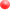Department of Mechanical Engineering

2.14 Analysis and Design of Feedback Control Systems

Fall Term 2003

Problem SetsProblem Set 1Problem Set 1 SolutionProblem Set 2 (due Sept 17)Problem Set 2 SolutionProblem Set 3 (due Sept. 24)Problem Set 3 SolutionProblem Set 4(due Oct. 1)Problem Set 4 SolutionProblem Set 5 (due Oct. 8)Problem Set 5 SolutionProblem Set 6 (due Oct. 22)Problem Set 6 SolutionProblem Set 7 (due Oct. 29)Problem Set 7 SolutionProblem Set 8 (due Nov. 5)Problem Set 8 SolutionProblem Set 9 (due Nov 24)Problem Set 10 (due Dec 8)